Custom SearchINDEFINITE INTEGRALS When we were finding the derivative of a function, we wrote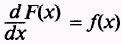where the derivative of F(x) is f(x). Our problem is to find F(x) when we are given f(x). We know that the symbol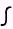... dx is the inverse of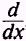or when dealing with differentials, the operator symbols d andare the inverse of each other; that is,and when the derivative of each side is taken, d annulling, we have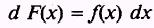or whereannuals, we have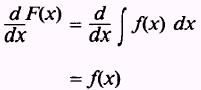From this, we find thatso that,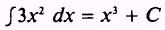Also we find that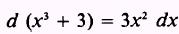so that,Again, we find that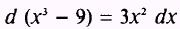so that,This is to say that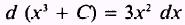and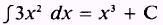where C is any constant of integration. A number that is independent of the variable of integration is called a constant of integration. Since C may have infinitely many values, then a differential expression may have infinitely many integrals differing only by the constant. This is to say that two integrals of the same function may differ by the constant of integration. We assume the differential expression has at least one integral. Because the integral contains C and C is indefinite, we call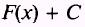an indefinite integral of f(x) dx. In the general form we sayWith regard to the constant of integration, a theorem and its converse are stated as follows: Theorem 1. If two functions differ by a constant, they have the same derivative. Theorem 2. If two functions have the same derivative, their difference is a constant.Integrated Publishing, Inc. - A (SDVOSB) Service Disabled Veteran Owned Small Business